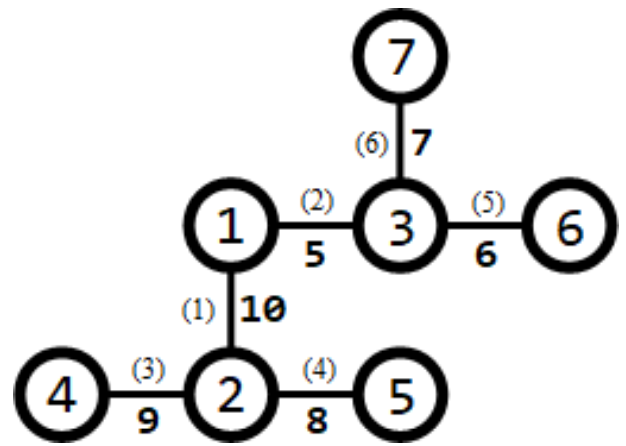# 洛谷 P5021 NOIp2018提高组 赛道修建 题解【二分答案】【贪心】【平衡树】

考场上因为平衡树的细节处理不过关而导致菊花图RE。丢了30分比较可惜。

## 题目描述

$\text{C}$城将要举办一系列的赛车比赛。在比赛前，需要在城内修建$m$条赛道。

$\text{C}$城一共有$n$个路口，这些路口编号为$1,2,\dots,n$，有$n-1$条适合于修建赛道的双向通行的道路，每条道路连接着两个路口。其中，第$i$条道路连接的两个路口编号为$a_i$和$b_i$，该道路的长度为$l_i$。借助这$n-1$条道路，从任何一个路口出发都能到达其他所有的路口。

【输入格式】

【输出格式】

【输入输出样例1】

 track.in track.out 7 1 1 2 10 1 3 5 2 4 9 2 5 8 3 6 6 3 7 7 31

【输入输出样例1说明】【输入输出样例2】

 track.in track.out 9 3 1 2 6 2 3 3 3 4 5 4 5 10 6 2 4 7 2 9 8 4 7 9 4 4 15

【输入输出样例2说明】1.经过第$1,6$条道路的赛道（从路口$1$到路口$7$），长度为$6+9=15$；
2.经过第$5,2,3,8$条道路的赛道（从路口$6$到路口$9$），长度为$4+3+5+4=16$；
3.经过第$7,4$条道路的赛道（从路口$8$到路口$5$），长度为$7+10=17$。

【数据规模与约定】

 测试点编号 $n$ $m$ $a_i=1$ $b_i=a_i+1$ 分支不超过$3$ 1 $\le 5$ $=1$ 否 否 是 2 $\le 10$ $\le n-1$ 是 3 $\le 15$ 是 否 否 4 $\le 1,000$ $=1$ 否 是 5 $\le 30,000$ 是 否 6 否 7 $\le n-1$ 是 8 $\le 50,000$ 9 $\le 1,000$ 否 是 是 10 $\le 30,000$ 11 $\le 50,000$ 12 $\le 50$ 否 13 14 $\le 200$ 15 16 $\le 1,000$ 17 否 18 $\le 30,000$ 19 20 $\le 50,000$

## 题解：

首先，对于每个题考虑需不需要开long long。答案最多是$m\times l_i=5\times 10^8$，因此不用开。

因为题目告诉我们，每个赛道都是一条链，并且不能重合，因此我们考虑使用合并的方式来找出这样的链，想到树形DP。

一个很简单的想法是，$f[i][j]$表示在$i$点凑出$j$条链的最短链最长可能长度，$g[i][j]$表示当$f[i][j]=1$时伸出的额外链长度。但是这里空间复杂度就达到了$O(n^2)$，不可取。我们反观题目，看到了想到可能可以二分，提示得仁至义尽。这个题合法与否是有单调性的没错，但是怎么判断是否存在一种方案，构建出$m$条长度不小于$x$的赛道呢？上面的树形DP显然在复杂度上就被淘汰了，剩下就只有贪心了。

在每个节点可以把每一棵子树中所能做出的最大贡献都拼出来，显然我们要找最合适的。也就是说，如果现在二分出来的数是$x$，找到一条链做出的贡献是$g_i$，就要找最小的另一个$g_j$满足$g_j\ge x-g_i$。那么此时在子树中匹配的对数就一定最多了，毕竟是两两匹配。此外，我们还要考虑是不是尽可能把所有的可以拼的都拼在一起。答案是肯定的。因为一旦能拼在一起，就能增加1的贡献，否则就算让这条链在外面做贡献，也最多只能造成1的影响，因此能在子树中拼就在子树中拼。

此时我们需要维护找$g_j$这一过程。可以二分，但是涉及到删数，对于菊花图来说，单次复杂度可能会被卡到$O(n^2)$，更不用说嵌套二分了。此时我用到了平衡树，不过需要注意的一点是，平衡树中可能同时出现多个权值相同的点，此时删数的编号有可能影响到后续的查询，那么我们给每个节点加上一个临时的编号就好了。而且需要先排序，然后从小到大枚举，这样留下尽可能大的数，可以为祖先造成较大的贡献。

时间复杂度为$O(n\log^2n)$。

## Code：

#include<cstdio>
#include<cstring>
#include<cstdlib>
#include<algorithm>
#include<vector>
using std::vector;
using std::sort;
{
int x=0,f=1;
char ch=getchar();
while(ch<'0'||ch>'9')
{
if(ch=='-')
f=-1;
ch=getchar();
}
while(ch>='0'&&ch<='9')
{
x=x*10+ch-'0';
ch=getchar();
}
return x*f;
}
struct node
{
int key,id,sz,rdm;
node *ls,*rs;
node(int key,int id)
{
this->key=key;
this->id=id;
rdm=rand();
sz=1;
ls=NULL;
rs=NULL;
}
node(){}
void maintain()
{
sz=(ls?ls->sz:0)+1+(rs?rs->sz:0);
}
}Node,*root;
int ncnt=-1;
node *Newnode(int x,int y)
{
Node[++ncnt]=node(x,y);
return &Node[ncnt];
}
node *Merge(node *a,node *b)
{
if(!a||!b)
return a?a:b;
if(a->rdm<b->rdm)
{
a->rs=Merge(a->rs,b);
a->maintain();
return a;
}
b->ls=Merge(a,b->ls);
b->maintain();
return b;
}
void split1(node *rt,int x,node *&a,node *&b)//按权值分裂
{
if(!rt)
{
a=NULL;
b=NULL;
return;
}
if(rt->key<=x)
{
a=rt;
split1(a->rs,x,a->rs,b);
}
else
{
b=rt;
split1(b->ls,x,a,b->ls);
}
rt->maintain();
return;
}
void split2(node *rt,int x,node *&a,node *&b)//按编号分裂
{
if(!rt)
{
a=NULL;
b=NULL;
return;
}
if(rt->id<=x)
{
a=rt;
split2(a->rs,x,a->rs,b);
}
else
{
b=rt;
split2(b->ls,x,a,b->ls);
}
rt->maintain();
return;
}
void Delete(int x)//删掉编号为x的点
{
node *a,*b,*c;
split2(root,x-1,a,b);
split2(b,x,b,c);
root=Merge(a,c);
}
int mn(node *rt)
{
if(rt->ls)
return mn(rt->ls);
return rt->id;
}
struct edge
{
int n,v,nxt;
edge(int n,int nxt,int v)
{
this->n=n;
this->nxt=nxt;
this->v=v;
}
edge(){}
}e;
void add(int from,int to,int v)
{
}
int mid,m;
int f,g;
bool used;
void dfs(int x,int from)
{
vector<int> t;//放在函数内的动态数组中
int cnt=-1;
f[x]=0,g[x]=0;
if(e[i].n!=from)
{
dfs(e[i].n,x);
g[e[i].n]+=e[i].v;
f[x]+=f[e[i].n];
if(g[e[i].n])
{
if(g[e[i].n]>=mid)
++f[x];
else
{
t.push_back(g[e[i].n]);
++cnt;
}
}
}
root=NULL;
ncnt=-1;//清空平衡树
sort(t.begin(),t.end());
for(int i=0;i<=cnt;++i)
{
used[i]=0;
root=Merge(root,Newnode(t[i],i));
}
for(int i=0;i<=cnt;++i)
if(!used[i])
{
Delete(i);
node *a,*b;
split1(root,mid-t[i]-1,a,b);
if(b)//如果有可以匹配的就匹配
{
used[i]=1;
int tmp=mn(b);
root=Merge(a,b);
Delete(tmp);
used[tmp]=1;
++f[x];
}
else
{
g[x]=g[x]>t[i]?g[x]:t[i];
root=Merge(a,b);
}
}
}
bool check()
{
memset(used,0,sizeof(used));
dfs(1,1);
return f>=m;//如果拼出m条以上的赛道即合法
}
int main()
{
int n,u,v,w;
for(int i=1;i<n;++i)
{
}
int l=0,r=500000000;
while(l<r)
{
mid=(l+r)>>1;
if(check())
l=mid+1;
else
r=mid;
}
printf("%d\n",l-1);
return 0;
}

### 1 说点什么0 Followers

Most reacted commentwpDiscuz